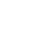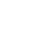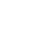# Acceleration

Physics is the science that studies motion and matter and how both work with forces and energy. It is a really big subject that has a lot of different “branches” of scientific study that include motion, sound, waves, electricity, light, sound, and even astronomy.

This science covers the tiniest particles and atoms all the way to the largest objects in the universe.

Two of the most famous scientists that helped to figure out the laws of physics are Sir Isaac Newton and Albert Einstein.

These scientists and others specialize in studying physics and have given us the understanding that all motion involves forces, acceleration, mass, energy, and work as the mechanics or “parts” of physics.

Everything in the universe is in constant motion, and the movement can be so little that you wouldn’t even know it all the way to incredibly fast.

Even though everything is constantly moving, the movement is almost always changing. Acceleration is a measurement of how fast something is moving in a specific amount of time.

If you are cruising along on a skateboard, enjoying the ride, the minute that you push off with your foot to make yourself go faster, you are changing the acceleration.

The same thing happens when the driver of a car pushes down on the gas pedal, making the car move forward faster. There is a change in the “velocity” that is called acceleration.

### Forces:

If you notice on the two examples that are given, a “force” was needed to change the acceleration. For the skateboard, it was a push from the other foot, and for the car it was pushing down on the gas pedal.

### Types of Acceleration:

Constant acceleration is a condition where an object is changing in acceleration at the same rate over time. When this happens, the object will go faster and faster, but the increase in “velocity” or speed is at the same rate.Freefall acceleration is where the force of gravity increases as an item falls making the object go faster as it falls. Gravity makes the object pick up speed.  On Earth, an object’s speed would also be affected by the friction of the air so that there is a point where the object can’t continue to increase in speed.

An example of this might be a skydiver. They jump from a plane and go faster and faster as they head downwards, but the friction of the air only lets them go so fast. This situation is called “terminal velocity.”

Average acceleration is where we measure the total velocity over time and then try to take an average.

This happens when forces alter the velocity and an object may go faster and then slow down. Think of a car speeding along on a highway and then getting stuck in a bit of a traffic jam. The acceleration is high when there isn’t any traffic, but low when sitting and waiting.

To get an average acceleration, you use math to figure out the average acceleration by dividing the change in velocity by the change in time:  Acceleration = (change in velocity)/(change in time)

### Deceleration or negative acceleration:

This is the slowing down of the acceleration or speed. This is what happens when you stop pushing down on the gas pedal or pushing off with your other foot on the skateboard. The velocity begins to slow down.

• Scientists have acknowledged that acceleration has units of measurement that are all its own. The measurements includes meters per second and the time is in seconds. The math formula for measuring acceleration is meters per second squared (m/s2).
• Acceleration can be positive when an object is moving faster or slower when an object is decreasing in velocity.
• Sir Isaac Newton created the second law of motion that says a force is always needed to produce acceleration and the larger the force, the faster the velocity change.

### Q&A:

What is the slowing down of acceleration called?
deceleration

What is always needed to produce acceleration?
a force

What is acceleration?
a measurement of how fast something is moving in a specific amount of time

What is constant acceleration?
a condition where an object is changing in acceleration at the same rate over time

Which scientist created the lawns of motion?
Sir Isaac Newton

What are the five mechanics or “parts” of physics?
forces, acceleration, mass, energy, and work

•History
•Science
•Geography
•Biography
•US History